GRE Subject Test: Chemistry : Acid-Base Reactions

Example Questions

← Previous 1

Example Question #1 : Acid Base Reactions

100mL of an unknown solution of NaOH is titrated with 3M HCl until neutralized. The resulting solution is evaporated, and 3.0g of white crystal are recovered. What was the concentration of the NaOH solution?

0.7M

1.0M

0.5M

0.3M

1.25M

0.5M

Explanation:

In the neutralization reaction between NaOH and HCl, NaCl salt is formed. When the solution is evaporated, this salt is left behind.

3.0g of NaCl is equivalent to 0.05mol NaCl. Since the titration is between a strong acid and a strong base, all of the NaOH in the original solution is converted to NaCl in a one-to-one ratio, meaning that mol NaCl = mol NaOH.

We now know that there was 0.05mol NaOH in the 100mL solution, so the concentration must have been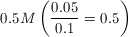.

Example Question #2 : Acid Base Reactions

What volume of 0.375M H2SO4 is needed to fully neutralize 0.5L of 0.125M NaOH?

41.7mL

167mL

83.3mL

0.5L

1.5L

83.3mL

Explanation:

This question requires use of the simple titration equation M1V1 = M2V2. The key is to identify that sulfuric acid has two equivalents of acidic hydrogens while NaOH has only one hydroxide equivalent. All wrong answer choices result from making this mistake or other calculation errors.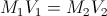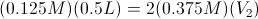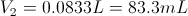Example Question #7 : Acid Base Chemistry

Consider the following reaction: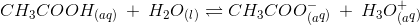Which of the following changes will increase the pH of this solution?

Increasing the pKa of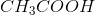Decreasing the volume of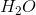Increasing the acetic acid concentration

Explanation:

To answer this question you need to use Le Chatelier’s principle. Adding sodium acetate to the solution will cause it to dissociate as follows: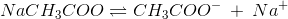The dissociation reaction will produce more acetate ions. According to Le Chatelier’s principle, the increase in acetate ions will shift the equilibrium of the reaction (given in the question) to the left. This means that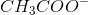and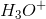will be utilized to form. This will cause a decrease in the amount of hydronium ions in solution. Recall that pH is increased when the concentration of hydrogen ions (or hydronium ions,) is decreased; therefore, adding sodium acetate will increase the pH of the solution.

Increasing acetic acid concentration will shift the equilibrium to the right and produce more hydronium ions, thereby decreasing the pH. Recall that you can never change the pKa of an acid. The pKa of acetic acid is around 4.75, and it cannot be altered. Le Chatelier’s principle only applies when there is a change in amount of aqueous or gaseous substances; liquid and solid substances will not shift the equilibrium. Changing the volume of liquid water will not change the concentration of hydronium ions.

Example Question #1 : Acid Base Reactions

Which of the following acids is polyprotic?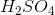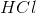None of these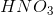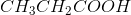Explanation:

Sulfuric acid () is considered a polyprotic acid because it has two ionizable protons in its molecular formula. The protons dissociate in an aqueous solution according to the acid-base equilibria below: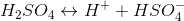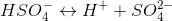All the other acids listed in the answer choices are monoprotic.

Example Question #1 : Acid Base Reactions

Which of the following acids is considered polyprotic?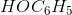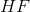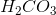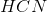All of theseExplanation:

Carbonic acid () is considered a polyprotic acid because it has two ionizable protons (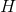atoms) in its molecular formula. The protons dissociate in an aqueous solution according to the acid-base equilibria below: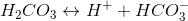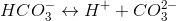The other acids are all monoprotic acids.

Example Question #4 : Acid Base Chemistry

Considering the Ka for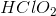is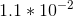, what is the Kb for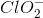?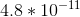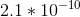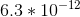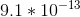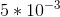Explanation:

The equilibrium governing the dissolution ofin water is: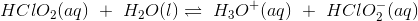is the conjugate acid of. In other words,is the conjugate base of.

Using the relationship,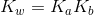, we can calculate the Kb.

Rearrange the equation and solve: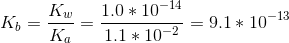Example Question #2 : Acid Base Reactions

Considering the Ka for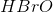is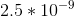, what is the Kb for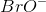?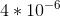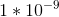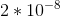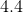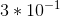Explanation:

The equilibrium governing the dissolution ofin water is: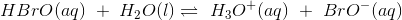is the conjugate acid of. In other words,is the conjugate base of.

Using the relationship,, we can calculate the Kb.

Rearrange the equation and solve: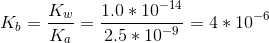Example Question #91 : Gre Subject Test: Chemistry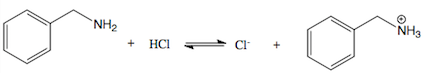Based on the equilibrium shown, what does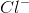act as?

An acid

A base

A catalyst

A cation

A base

Explanation:

A base is a substance that can accept a proton. The conjugate base of an acid is formed when the acid donates a proton. In this case,is the conjugate base to the acid. This is becausedonates a hydrogen ion to the organic molecule to form, the conjugate base.

Example Question #1 : Acid Base Reactions

Which of the following is the weakest acid?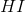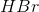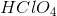Explanation:(hydrofluoric acid) is the weakest acid. Fluoride ion is the most electronegative ion. Among the other halogens, its atomic radius is smaller, and therefore bonds more strongly with hydrogen and therefore does not completely dissociate in solution as compared to,, and. Perchloric acid is a strong acid and dissociates completely in solution.

Example Question #4 : Acid Base Reactions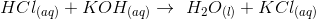What is the type of reaction given?

Neutralization Reaction

Sublimation Reaction

Synthesis Reaction

Redox Reaction

Catalytic reaction